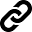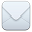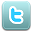SHARE:## sectionB

Variable Question text Label
b001_aOn a scale from 0 to 100, where 0 means absolutely no chance and 100 means absolutely certain, what is the percent chance that your average annual earnings from work ^FLRelevantAge[cnt] will be less than \$^X_[cnt, 1]?
b001_bWhat is the percent chance that your average annual earnings ^FLRelevantAge[cnt] will be less than \$^X_[cnt,2]?
b001_cWhat is the percent chance that your average annual earnings ^FLRelevantAge[cnt] will be less than \$^X_[cnt,3]?
b001_dWhat is the percent chance that your average annual earnings ^FLRelevantAge[cnt] will be less than \$^X_[cnt,4]?
b002_aOn a scale from 0 to 100, where 0 means absolutely no chance and 100 means absolutely certain, what is the percent chance that your average annual earnings from work ^FLRelevantAge[cnt] will be less than \$^X_[cnt,4]?
b002_bWhat is the percent chance that your average annual earnings from work ^FLRelevantAge[cnt] will be less than \$^X_[cnt,3]?
b002_cWhat is the percent chance that your average annual earnings from work ^FLRelevantAge[cnt] will be less than \$^X_[cnt,2]?
b002_dWhat is the percent chance that your average annual earnings from work ^FLRelevantAge[cnt] will be less than \$^X_[cnt,1]?
b003_aOn a scale from 0 to 100, where 0 means absolutely no chance and 100 means absolutely certain, what is the percent chance that your average annual earnings from work ^FLRelevantAge[cnt] will be less than \$^X_[cnt,1]?
b003_bOn a scale from 0 to 100, where 0 means absolutely no chance and 100 means absolutely certain, what is the percent chance that your average annual earnings from work ^FLRelevantAge[cnt] will be less than \$^X_[cnt,2]?
b003_cOn a scale from 0 to 100, where 0 means absolutely no chance and 100 means absolutely certain, what is the percent chance that your average annual earnings from work ^FLRelevantAge[cnt] will be less than \$^X_[cnt,3]?
b003_c_minb003_c min
b003_dOn a scale from 0 to 100, where 0 means absolutely no chance and 100 means absolutely certain, what is the percent chance that your average annual earnings from work ^FLRelevantAge[cnt] will be less than \$^X_[cnt,4]?
b003_d_minb003_d min
b004_aOn a scale from 0 to 100, where 0 means absolutely no chance and 100 means absolutely certain, what is the percent chance that your average annual earnings from work ^FLRelevantAge[cnt] will be less than \$^X_[cnt,4]?
b004_bOn a scale from 0 to 100, where 0 means absolutely no chance and 100 means absolutely certain, what is the percent chance that your average annual earnings from work ^FLRelevantAge[cnt] will be less than \$^X_[cnt,3]?
b004_b_maxb004_b max
b004_cOn a scale from 0 to 100, where 0 means absolutely no chance and 100 means absolutely certain, what is the percent chance that your average annual earnings from work ^FLRelevantAge[cnt] will be less than \$^X_[cnt,2]?
b004_c_maxb004_c max
b004_dOn a scale from 0 to 100, where 0 means absolutely no chance and 100 means absolutely certain, what is the percent chance that your average annual earnings from work ^FLRelevantAge[cnt] will be less than \$^X_[cnt,1]?
b004_d_maxb004_d max